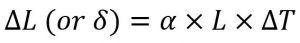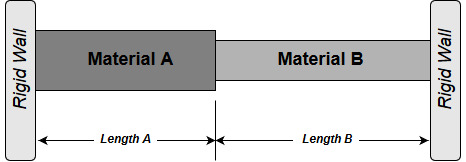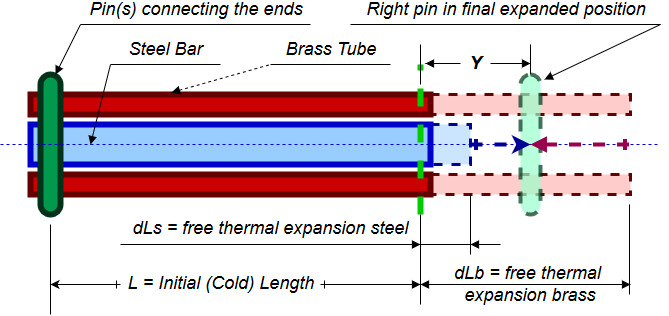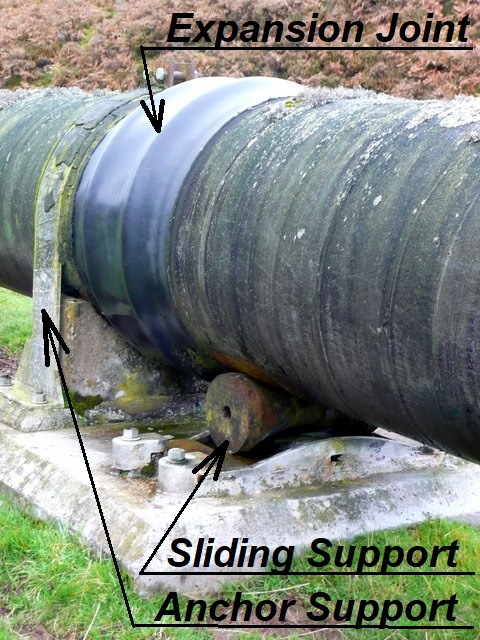Main Body

# Thermal Expansion Stress

Thermal Expansion

### Learning Objectives

At the end of this section you should be able to calculate problems involving

• Unrestricted thermal expansion
• Restricted thermal expansion

All materials subject to a temperature change will expand or contract proportional with their length and temperature difference.  Some materials will expand or contract more than others; the qualitative property that indicates how much will they expand is known as the Linear Thermal Expansion Coefficient (α), measured in m/(m ºC) or (in/in ºF).  Also units like 1/ºC or 1/ºF can be used.

The change in length due to thermal expansion is calculated with:where δ is the change in length, L is the original length (makes sure both are in the same units) and ΔT is the temperature difference.

For example if steel has a thermal expansion coefficient of 11.7×10-6 1/ºC it means that a 1 m long bar subject to a temperature increase of 1ºC will expand 11.7×10-6 m, or 0.0117 mm.  This may seem like a negligible amount but if you consider a steam pipe of 50 m long installed at 12ºC and operating at 212ºC (2000 kPa saturation pressure), the thermal expansion would be equivalent to 11.7 cm, or an equivalent strain of 0.002.  This is very important for the piping designers because they have to allow for this expansion or factor it in the stress calculations.

Volumetric thermal expansion of solids (isotropic materials) is calculated in a similar way using (3×α) as expansion coefficient.  When calculating liquids volumetric expansion, the volumetric expansion coefficient is β, with typical values as listed in The Engineering Toolbox.

Pipelines expansion

Typically pipelines are relatively long and may see a significant temperature increase between installation and operating temperatures.  As result, high magnitude thermal expansion stresses may develop if the supports are not adequately designed.  In addition to that, the expansion of the pipe increases the load on machinery and vessels nozzles.

### Pipe cold springing

There is an abundance of articles and discussions on this topic in piping design groups, easily accessible through an internet search using key word strings “pipe cold springing” or “pipe cold pull“; it is also addressed in ASME B31.3.

“Pipe cold springing is defined as the process of intentional deformation (usually accomplished by cutting short or long the pipe runs between two anchors) of piping during assembly to produce a desired initial displacement and stress.  It is also defined as the intentional stressing and elastic deformation of the piping system during the erection cycle to permit the system to attain more favorable reactions and stresses in the operating condition.” 

Operating engineers are advised to be familiar with this practice since it may be used in steam pipes.  There have been circumstances when hired contractors when disassembling steam lines complained about lines not being fitted properly; the pipes would sprung back when unbolted.  Avoid costly repairs and unnecessary alterations by being familiar with this procedure and by knowing your plant.

Thermal stresses in composite bars

“The composite tube consists of two different alloys metallurgically bonded together to achieve good thermal transfer properties. One alloy is used to withstand corrosion, while the other is often an approved pressure vessel material.

Typical applications for composite tubes are steam boilers with corrosive conditions, such as:

• Black liquor recovery boilers (BLRB)
• Syngas coolers
• Waste heat boilers
• Waste-to-energy boilers

Composite tube (compound tube) are suitable for applications where the conditions outside and inside the tube require material properties that cannot be met by one material only.” 

While Power Engineering students may not see a direct application of these principles, the following types of problems are part of their 2nd and 1st class curriculum.

## Case A

The following diagram represent a typical restricted thermal expansion scenario, with compound bars:Given all the materials properties and dimensions, the objective is to calculate the stress in each section when the temperature is increased by a given ΔT.

When the bars are heated, each will attempt tend to expand equivalent to their unrestricted ΔL.  Given that the expansion is restricted, each bar will be subject to compression which in turn generates compression stresses.  The sum of the two corresponding (yet imaginary) compression deformations will be equal to the sum easily quantifiable unrestricted thermal expansions.  Furthermore, considering that the forces applied by each bar are the same (static/balanced system), this compression force can easily be calculated.   Factoring in the cross-sectional areas of each bar leads to finding the stress developed in each material.

## Case B

In the second scenario, a bar is pinned at both ends inside a tube of a different material.  When heated up, one material would expand freely more than the other.  The lower expansion material will be pulled outwards in tension by the second one that attempts to expand more.  In turn, the material that would freely expand more it is pulled in (compression) by the material that expands less.  See the following figure for clarification.Objective of this exercise is to find the stresses generated in each material.  The approach at solving this problem is as follows.

• When heated up, the brass tube is pulled by the steel rod, generating a compression stress in the tube.  The brass tube restricted deformation will be (dLb – Y), where Y is the actual combined deformation of the composite bar.
• Similarly, the steel bar is pulled out by the brass tube, generating a tensile stress.  The actual (restricted) deformation of the steel bar is (Y-dLs).
• Note than in the above dL is the free thermal expansion of each material.
• From the diagram, (dLb – Y) + (Y – dLs) = dLb – dLs
• Substitute in the above dL=α×L×ΔT for each material, divide equation by L = initial length and find:
• ε brass + ε steel = (α brass – α steel) × ΔT
• Substitute E = σ / ε in the above for each material and the resulting equation represents a relation between the stress in each material, function only of the known elastic/thermal expansion properties and the temperature difference.
• brass / E brass) + (σ steel / E steel) = (α brass – α steel) × ΔT     (eqn. B1)
• The second equation comes from the observation that outward pull force of the brass is equal to the inward pull of the steel.  This can be expressed as:
• σ brass × A brass = σ steel × A steel                                                (eqn. B2)
• Solve equation B2 for σ brass and substitute into equation B1.  Solve equation B1 for α steel and your final result is dependent only on materials properties, cross-sections and temperature difference.
• Once σ steel answer is found, go back to equation B2 and find σ brass.
• This may appear as a math/algebra demanding problem, and it is; however, it is a classical problem with a standard solution which means that every question will be solve following the same approach.

### Assigned Problems

When solving the following problems find the required data in the textbook appendices, provided external resources or other reputable sources; always quote the source.

Problem 1: A firetube boiler is powered using bunker fuel oil.   The storage tank is of an open top construction, 2 m diameter and 3 m height. Oil is added when the ambient temperature is 10ºC.   During start-up the temperature suddenly rises to 35ºC.   How many centimeters below the tank top can you fill in the tank, so that you maximize the oil volume while avoiding any spillage?  Coefficient of linear expansion of tank material is 12×10-6 /ºC and volumetric expansion coefficient for oil is 9×10-4 /ºC.

Problem 2: During installation, a turbine casing bolt is heated to 250ºC and the nut is tightened so that no stress is produced (hand-tight).  When it cools down to the operation temperature of 50ºC the bolt adequately secures the assembly.  Determine the tensile stress and strain in the bolt and the force carried by the bolt.  The bolt effective length is 300 mm, diameter 50 mm and Ebolt = 200 GPa.

Problem 3: A new above ground pipeline will transport crude oil from Northern Alberta, south.  To compensate for thermal expansion each straight section of the pipeline will be equipped with corrugated expansion joints that allow 23 mm axial expansion and 18 mm axial compression (figure).   The pipeline will be installed early summer when ambient temperatures may conservatively assume to be 23ºC.  The pipe is DN 600 Sch 40 and Carbon Steel material.

Determine the maximum straight pipe length between two anchor points (in m) for extreme Alberta temperatures while assuming that due to bush-fires the pipe metal temperature can reach as high as 100ºC.   For your specified pipe length what would be the maximum stress developed in the material if the thermal expansion is restricted?Problem 4: An 8″ Schedule 40 straight length of steam pipe is fitted between two fixed anchor supports with no allowance for expansion.   If the compressive stress in the pipe must be limited to 50.7 ksi when in operation at 430ºF, determine the initial tensile stress that must be applied during installation at 60ºF.  What equivalent tensile force is required by this cold-springing installation?

Problem 5: A single-pass double pipe heat exchanger is constructed using 1″ nominal thickness ASTM B88 Type K Copper tubing for the internal tube and 2″ nominal size, medium wall thickness, steel tubing for the external shell.  The length of the heat exchanger is 24″.  The heat exchanger is assembled stress free at 20ºC but in operation the tubes wall temperatures reach 120ºC.   Determine the stresses generated by thermal expansion in both, steel and copper tubing.  Use:

Problem 6: Recommend one improvement to this chapter.